# Bode Plot, Gain Margin and Phase Margin (Plus Diagrams)

Contents

## What is a Bode Plot

A Bode plot is a graph commonly used in control system engineering to determine the stability of a control system. A Bode plot maps the frequency response of the system through two graphs – the Bode magnitude plot (expressing the magnitude in decibels) and the Bode phase plot (expressing the phase shift in degrees).

Bode plots were first introduced in the 1930s by Hendrik Wade Bode while he was working at Bell Labs in the United States. Although Bode plots offer a relatively simple method to calculate system stability, they can not handle transfer functions with right half plane singularities (unlike Nyquist stability criterion).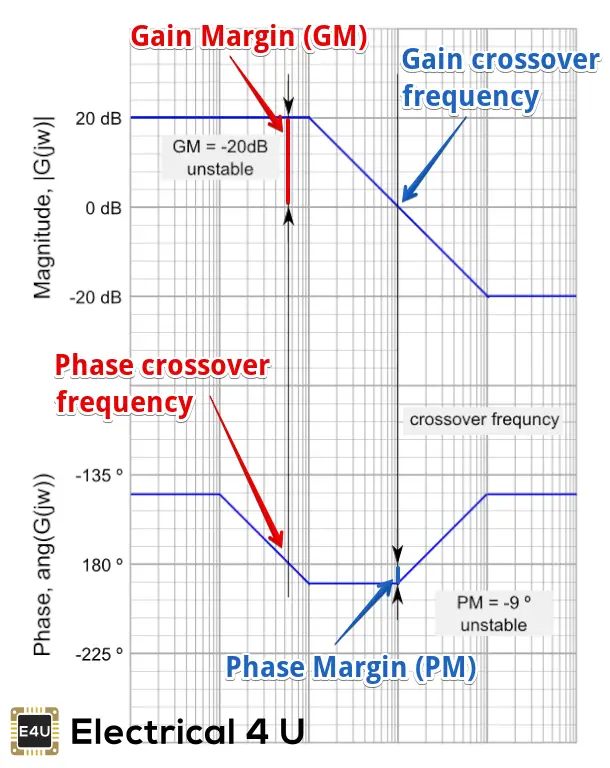The Gain Margin and Phase Margin shown on a Bode Plot

Understanding gain margins and phase margins are crucial to understanding Bode plots. These terms are defined below.

## Gain Margin

The greater the Gain Margin (GM), the greater the stability of the system. The gain margin refers to the amount of gain, which can be increased or decreased without making the system unstable. It is usually expressed as a magnitude in dB.

We can usually read the gain margin directly from the Bode plot (as shown in the diagram above). This is done by calculating the vertical distance between the magnitude curve (on the Bode magnitude plot) and the x-axis at the frequency where the Bode phase plot = 180°. This point is known as the phase crossover frequency.

It is important to realize that the Gain and the Gain Margin are not the same things. In fact, the Gain Margin is the negative of the gain (in decibels, dB). This will make sense when we look at the Gain margin formula.

### Gain Margin Formula

The formula for Gain Margin (GM) can be expressed as: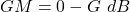Where G is the gain. This is the magnitude (in dB) as read from the vertical axis of the magnitude plot at the phase crossover frequency.

In our example shown in the graph above, the Gain (G) is 20. Hence using our formula for gain margin, the gain margin is equal to 0 – 20 dB = -20 dB (unstable).

## Phase Margin

The greater the Phase Margin (PM), the greater will be the stability of the system. The phase margin refers to the amount of phase, which can be increased or decreased without making the system unstable. It is usually expressed as a phase in degrees.

We can usually read the phase margin directly from the Bode plot (as shown in the diagram above). This is done by calculating the vertical distance between the phase curve (on the Bode phase plot) and the x-axis at the frequency where the Bode magnitude plot = 0 dB. This point is known as the gain crossover frequency.

It is important to realize that the phase lag and the Phase Margin are not the same things. This will make sense when we look at the phase margin formula.

### Phase Margin Formula

The formula for Phase Margin (PM) can be expressed as: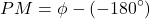Whereis the phase lag (a number less than 0). This is the phase as read from the vertical axis of the phase plot at the gain crossover frequency.

In our example shown in the graph above, the phase lag is -189°. Hence using our formula for phase margin, the phase margin is equal to -189° – (-180°) = -9° (unstable).

As another example, if an amplifier’s open-loop gain crosses 0 dB at a frequency where the phase lag is -120°, then the phase lag -120°. Hence the phase margin of this feedback system is -120° – (-180°) = 60° (stable).

## Bode Plot Stability

Below is a summarized list of criterion relevant to drawing Bode plots (and calculating their stability):

1. Gain Margin: Greater will the gain margin greater will be the stability of the system. It refers to the amount of gain, which can be increased or decreased without making the system unstable. It is usually expressed in dB.
2. Phase Margin: Greater will the phase margin greater will be the stability of the system. It refers to the phase which can be increased or decreased without making the system unstable. It is usually expressed in phase.
3. Gain Crossover Frequency: It refers to the frequency at which the magnitude curve cuts the zero dB axis in the bode plot.
4. Phase Crossover Frequency: It refers to the frequency at which phase curve cuts the negative times the 180o axis in this plot.
5. Corner Frequency: The frequency at which the two asymptotes cuts or meet each other is known as break frequency or corner frequency.
6. Resonant Frequency: The value of frequency at which the modulus of G (jω) has a peak value is known as the resonant frequency.
7. Factors: Every loop transfer function {i.e. G(s) × H(s)} product of various factors like constant term K, Integral factors (jω), first-order factors ( 1 + jωT)(± n) where n is an integer, second-order or quadratic factors.
8. Slope: There is a slope corresponding to each factor and slope for each factor is expressed in the dB per decade.
9. Angle: There is an angle corresponding to each factor and angle for each factor is expressed in the degrees.

Now there are some results that one should remember in order to plot the Bode curve. These results are written below:

• Constant term K: This factor has a slope of zero dB per decade. There is no corner frequency corresponding to this constant term. The phase angle associated with this constant term is also zero.
• Integral factor 1/(jω)n: This factor has a slope of -20 × n (where n is an integer)dB per decade. There is no corner frequency corresponding to this integral factor. The phase angle associated with this integral factor is -90 × n. Here n is also an integer.
• First order factor 1/ (1+jωT): This factor has a slope of -20 dB per decade. The corner frequency corresponding to this factor is 1/T radian per second. The phase angle associated with this first factor is -tan– 1(ωT).
• First order factor (1+jωT): This factor has a slope of 20 dB per decade. The corner frequency corresponding to this factor is 1/T radian per second. The phase angle associated with this first factor is tan– 1(ωT) .
• Second order or quadratic factor : [{1/(1+(2ζ/ω)} × (jω) + {(1/ω2)} × (jω)2)]: This factor has a slope of -40 dB per decade. The corner frequency corresponding to this factor is ωn radian per second. The phase angle associated with this first factor is

## How to Draw Bode Plot

Keeping all the above points in mind, we are able to draw a Bode plot for any kind of control system. Now let us discuss the procedure of drawing a Bode plot:

1. Substitute the s = jω in the open loop transfer function G(s) × H(s).
2. Find the corresponding corner frequencies and tabulate them.
3. Now we are required one semi-log graph chooses a frequency range such that the plot should start with the frequency which is lower than the lowest corner frequency. Mark angular frequencies on the x-axis, mark slopes on the left hand side of the y-axis by marking a zero slope in the middle and on the right hand side mark phase angle by taking -180o in the middle.
4. Calculate the gain factor and the type of order of the system.
5. Now calculate slope corresponding to each factor.

For drawing the Bode magnitude plot:

• Mark the corner frequency on the semi-log graph paper.
• Tabulate these factors moving from top to bottom in the given sequence.
1. Constant term K.
2. Integral factor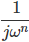3. First order factor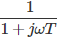4. First order factor (1+jωT).
5. Second order or quadratic factor:
• Now sketch the line with the help of the corresponding slope of the given factor. Change the slope at every corner frequency by adding the slope of the next factor. You will get the magnitude plot.
• Calculate the gain margin.

For drawing the Bode phase plot:

1. Calculate the phase function adding all the phases of factors.
2. Substitute various values to the above function in order to find out the phase at different points and plot a curve. You will get a phase curve.
3. Calculate the phase margin.

## Bode Stability Criterion

Stability conditions are given below:

1. For a Stable System: Both the margins should be positive or phase margin should be greater than the gain margin.
2. For Marginal Stable System: Both the margins should be zero or phase margin should be equal to the gain margin.
3. For Unstable System: If any of them is negative or phase margin should be less than the gain margin.

## Advantages of a Bode Plot

1. It is based on the asymptotic approximation, which provides a simple method to plot the logarithmic magnitude curve.
2. The multiplication of various magnitude appears in the transfer function can be treated as an addition, while division can be treated as subtraction as we are using a logarithmic scale.
3. With the help of this plot only we can directly comment on the stability of the system without doing any calculations.
4. Bode plots provide relative stability in terms of gain margin and phase margin.
5. It also covers from low frequency to high frequency range.

Want To Learn Faster? 🎓
Get electrical articles delivered to your inbox every week.
No credit card required—it’s 100% free.